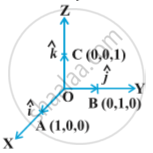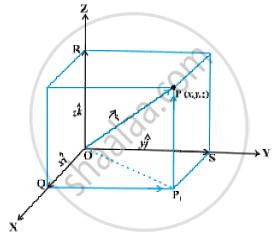Advertisement Remove all ads

# Components of a Vector

Advertisement Remove all ads

#### notes

Let us take the points A(1, 0, 0), B(0, 1, 0) and C(0, 0, 1) on the x-axis, y-axis and z-axis, respectively. Then, clearly
|vec (OA)| = 1, |vec (OB)| = 1 and |vec (OC)| = 1The vectors vec (OA) , vec (OB) and vec (OC), each having  magnitude 1, are called unit vectors along the axes OX, OY and OZ, respectively, and denoted by hat i , hat j ,and hat k  respectively.
Now, consider the position vector vec (OP) of a point P(x, y, z) as in following Fig . Let P_1 be the foot of the perpendicular from P on the plane XOY.We, thus, see that P_1P is parallel to z-axis. As hat i,hat j and hat k are the unit vectors along the x, y and z-axes, respectively, and by the definition of the coordinates of P, we have vec (P_1P) = vec (OR) = z hatk . Similarly, vec (QP_1) = vec (OS) = y hat j and vec (OQ) = x hat i .

Therefore, it follows that vec (OP_1) = vec (OQ )+ vec (QP_1) = x hat i + y hat j
and vec (OP) = vec (OP_1) + vec (P_1P) = x hat i + y hat j + z hat k
Hence, the position vector of P with reference to O is given by
vec (OP) (or vec r) = x hat i + y hat j + zhat k
This form of any vector is called its component form. Here, x, y and z are called as the scalar components of  vec r, and x hat i, y hat j and z hat k are called the vector components of vec r along the respective axes. Sometimes x, y and z are also termed as rectangular components.

The length of the vector vec r = x hat i + y hat j + z hat k , is readily determined by applying the Pythagoras theorem twice. We note that in the right angle triangle OQP_1 in above fig.

|vec (OP_1)| = sqrt | vec (OQ)|^2 + |vec (QP_1)|^2 = sqrt (x^2 + y^2),

and in the right angle triangle OP_1P, we have

vec (OP) = sqrt | vec (OP_1)|^2 + |vec (P_1P)|^2 = (sqrt (x^2 + y^2)+z_2),

Hence, the length of any vector vec r = x hat i + y hat j + z hat k is given by
|vec r| = |xhat i + y hat j +z hat k| = sqrt (x^2 + y^2 + z^2)
If vec a and vec b  are any  two vectors given in the component form a_1hat i + a_2hat j + a_3hat k and b_1hat i + b_2hat j + b_3hat k respectively , then

(i) the sum (or resultant) of the vectors vec a "and" vec b  is given by
vec a + vec b = (a_1 + b_1) hat i + (a_2 + b_2) hat j + (a_3 + b_3) hat k

(ii) the difference of the vector vec a    and    vec b is given by
vec a - vec b = (a_1 - b_1) hat i + (a_2 - b_2) hat j + (a_3 - b_3) hat k

(iii) the vectors vec a and vec b are equal if and only if
a_1 =b_1,  a_2 = b_2   and  a_3 = b_3

(iv) the multiplication of vector vec a by any scalar λ is given by
lambda vec a = (lambda a_1)hat i + (lambda a_2) hat j + (lambda a_3) hat k

The addition of vectors and the multiplication of a vector by a scalar together give the following distributive laws:
Let vec a and vec b be any two vectors, and k and m be any scalars. Then
i) k vec a + m vec a = (k+m)vec a
ii) k(m vec a) = (km) vec a
iii) k(vec a + vec b) = k vec a + k vec b
Video link : https://youtu.be/hBlkiMDiBNI

If you would like to contribute notes or other learning material, please submit them using the button below.

#### Video Tutorials

We have provided more than 1 series of video tutorials for some topics to help you get a better understanding of the topic.

Series 1

Series 2

### Shaalaa.com

Components of Vector [00:25:15]
S
##### Series: 1
0%

Advertisement Remove all ads
Share
Notifications

View all notifications

Forgot password?